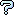All about flooble | fun stuff | Get a free chatterbox | Free JavaScript | Avatarsperplexus dot infoTwo Digit Average 2 (Posted on 2020-04-15)Part 1:

There is a two digit number with the unique property that if a decimal point is inserted between the two digits, the resulting number is the average of the square of the digits.

Part 2:

There is a four digit number with the unique property that if a decimal point is inserted between the first and second digits, the resulting number is the average of the square of the digits.

What are these averages? Report your answers with the decimal point appropriately positioned, and include any trailing zeros, if any, to clearly indicate the number of digits present.

Bonus: What about raising digits to integer powers higher than 2, different numbers of digits, and other locations of the decimal point? How many can you find?

 No Solution Yet Submitted by Larry No RatingComments: ( Back to comment list | You must be logged in to post comments.)Yes, but there's still the Bonus Comment 2 of 2 |Bonus:
But you'll probably need a program to find solutions for exponents higher than 2, numbers of digits other than 4, and other decimal point locations.
For example 4 digits, power of 2, and decimal point between the 2nd and 3rd digits.
Or 6 digits, power of 4, decimal anywhere.

 Posted by Larry on 2020-04-16 10:10:01Please log in:

 Search: Search body:
Forums (2)